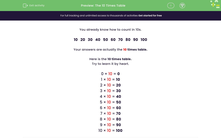# The 10 Times Table

In this worksheet, students answer questions on the 10 times table. Counting in 10s may help.Key stage:  KS 1

Curriculum topic:   Number: Multiplication and Division

Curriculum subtopic:   Solve Multiplication/Division Problems

Difficulty level:#### Worksheet Overview

You already know how to count in 10s.

10   20   30   40   50   60   70   80   90   100

Here is the 10 times table.

Try to learn it by heart.

0 × 10 = 0

1 × 10 = 10

2 × 10 = 20

3 × 10 = 30

4 × 10 = 40

5 × 10 = 50

6 × 10 = 60

7 × 10 = 70

8 × 10 = 80

9 × 10 = 90

10 × 10 = 100

### What is EdPlace?

We're your National Curriculum aligned online education content provider helping each child succeed in English, maths and science from year 1 to GCSE. With an EdPlace account you’ll be able to track and measure progress, helping each child achieve their best. We build confidence and attainment by personalising each child’s learning at a level that suits them.

Get started